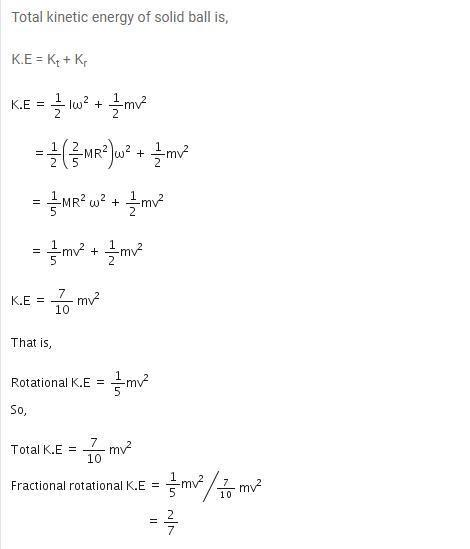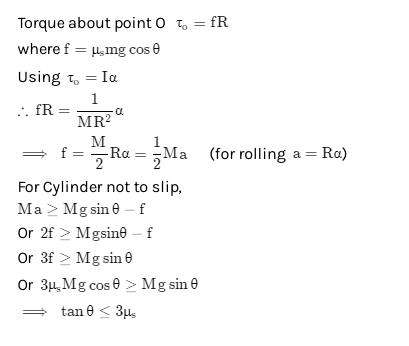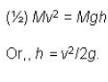Courses

# Test: Rolling Motion

## 10 Questions MCQ Test Physics Class 11 | Test: Rolling Motion

Description
This mock test of Test: Rolling Motion for JEE helps you for every JEE entrance exam. This contains 10 Multiple Choice Questions for JEE Test: Rolling Motion (mcq) to study with solutions a complete question bank. The solved questions answers in this Test: Rolling Motion quiz give you a good mix of easy questions and tough questions. JEE students definitely take this Test: Rolling Motion exercise for a better result in the exam. You can find other Test: Rolling Motion extra questions, long questions & short questions for JEE on EduRev as well by searching above.
QUESTION: 1

### A spherical solid ball rolls on a table. What fraction of its total K.E. is rotational K.E. ?

Solution:QUESTION: 2

### A body is rolling down an inclined plane without slipping. How does the acceleration of the rolling body depend on its radius?

Solution:

The acceleration of a rolling body is independent of its mass and radius.

QUESTION: 3

### Acceleration (a) of a body of radius R rolling down an inclined plane of inclination is given by

Solution:
QUESTION: 4

What is the moment of inertia of a solid sphere about its diameter?

Solution:

We have to consider solid sphere contribution of infinite small solid cylinder.
Icylinder = ½ MR²
dI = ½ dmr²
dm = pdV
dV = πr²dx
By substituting dV into dm
dm = pπr²dx
Now dm into dI
dI = ½ r²pπr²dx = ½ pπr⁴dx
Now, r² = R²-x²
dI = ½ pπ(R²-x²)²dx
Integrate both sides
I = ½ pπ 16/15 R²
P = M/V
P = M/4/3πR³ (density of sphere)
After substituting
I = 2/5MR2

QUESTION: 5

What is the coefficient of friction when a cylinder rolls down without slipping on an inclined plane?

Solution:QUESTION: 6

If a solid cylinder rolls up along a inclined plane with an initial velocity v. It will rise up to a height h equal to

Solution:
QUESTION: 7

What will be the speed of center of mass, if a solid sphere of radius 20 cm which rotates at 2 rps along a straight line?

Solution:

V = rw
V = 20 × 10-2 × 2π(2)
V = 2.51 m/s

QUESTION: 8

Two discs A and B are mounted on a vertical axle. Suppose the discs have moment of inertia as I and 2 I respectively about an axis. Disc A is given angular velocity ω, using the potential energy of a spring compressed by x1. Similarly the potential energy of a spring compressed by x2 gives an angular velocity of 2ω to Disc B. Considering that both the discs rotate in same direction .Find the ratio x1/ x2 :

Solution:
QUESTION: 9

A small cylinder rolling with a velocity v along a horizontal surface encounters a smooth inclined surface. The height ‘h’ up to which the cylinder will ascend is

Solution:

A body can roll along a surface only if the surface is rough. The body will roll up to the foot of the inclined smooth surface. It will continue to spin with the angular speed it has acquired, and will slide up to a certain height, maintaining its spin motion throughout the smooth surface. Its translational kinetic energy alone is responsible for its upward motion along the smooth incline so that the height up to which it will rise is given byQUESTION: 10

The kinetic energy of a rolling wheel is

Solution:

The total KE is the sum of translational KE and rotational KE.